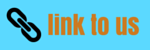# Structure of atoms class9 worksheet

Given below are the CBSE Structure of atoms class9 worksheet
a) Concepts questions
b) Calculation problems
c) Multiple choice questions
d) Long answer questions
e) Fill in the blank's

## Fill in the blanks:

1. According to Maharishi Kanad, the tiniest to tiny particle of a pure substance is called _________.
2. An atom is the smallest unit of an element which takes part in a _________.
3. Mass of an electron is 1/1837 times less than the mass of one atom of__________.
4. The K-shell of any atom cannot have more than _________ electrons.
5. Isotopes are the atoms of ___________ element, having same atomic number but different mass number.
6. An atom of an element has 11 protons 11 electrons and 12 neutrons. The atomic mass of the atom is __________.
7. Almost all the mass of an atom is concentrated in a small region of space called the _______.
8. The subatomic particle not present in a hydrogen atom is________.
9. Cathode ray are a beam of fast moving ______ .
10. ________and________ more or less complexly make up the mass of an atom.
11. The number of neutrons in the neutrons in the neutrons of an atom can be calculated by _______ the atomic number of _______its mass number.
12. The isotopes of an element do not differ in the number of _______ but do differ in the number of _______.
13. The type of radiations, which are not deflected in presence of electric or magnetic field are termed as rays.
14. The penetrating power of B-rays is_______ times more than a-rays.
15. Synthetic elements are those which are prepared by _________.
16. The control rods in a nuclear reactor are made up of _________.
17. _______ are stored in deep mines which are not in use.
18. When an elements emits B-particle, its mass number________.
19. Y-rays are________ radiations.
20. The difference between the actual isotopic mass and the sum of masses of protons, neutrons and electrons is called_________.
21. _______ are the best dombarding particles.
22. ________ is used for the treatment of leukaemia.

## True / False:

1. Radioactive isotope of iodine is used for making the medicine called tincture iodne.
2. Thomson proposed that the nucleus of an atom contains protons and neutrons.
3. J.J. Thomson proposed that the nucleus of an atom contains only nucleons.
4. An electron has a mass that is much less than a proton.
5. There is no particle of matter smaller than an atom.
6. Atoms of an element may have more or less neutrons or electrons than other atoms of the same element.
7. The innermost atomic shell can hold a maximum of 18 electons.
8. B- Particles are fast moving electrons carrying negative charge.
9. Nuclear chemistry has nothing to do with the electrons present in an atom.
10. B- particles are nothing but electrons.
11. a-particles are same thing as helium atoms.
12. Out of a, B and y- rays travel with the highest speed and have highest ionizing power.
13. A loss of B-particle result n the production of isobars.
14. There is no difference between ‘artificial radioactivity’ and ‘induced radioactivity’.
15. Iodine- 131 is used in the treatment of thyroid disorders.
16. In a given electric field, B-particles are deflected more than a- particles in spite of a- particles having larger charge.